# Various examples in Basis Path Testing

• Difficulty Level : Expert
• Last Updated : 10 Jul, 2020

Prerequisite – Basis Path Testing
We have seen the steps involved in designing the test cases for a program using the basis path testing in the previous article. Now, let’s solve an example following the same steps.

Question : Consider the given program that checks if a number is prime or not. For the following program :

1. Draw the Control Flow Graph
2. Calculate the Cyclomatic complexity using all the methods
3. List all the Independent Paths
4. Design test cases from independent paths

 `int` `main()``{``    ``int` `n, index;``    ``cout << ``"Enter a number: "` `<< endl;``    ``cin >> n;``    ``index = 2;``    ``while` `(index <= n - 1) {``        ``if` `(n % index == 0) {``            ``cout << ``"It is not a prime number"` `<< endl;``            ``break``;``        ``}``        ``index++;``    ``}``    ``if` `(index == n)``        ``cout << ``"It is a prime number"` `<< endl;``} ``// end main`

Solution :
1. Draw the Control Flow Graph –

• Step-1:
Start numbering the statements after declaration of the variables (if no variables have been initialized in that statement). However, if a variable has been initialized and declared in the same line, then numbering should start from that line itself.

For the given program, this is how numbering will be done:

```int main()
{
int n, index;
1    cout << "Enter a number: " <> n;
3    index = 2;
4    while (index <= n - 1)
5    {
6        if (n % index == 0)
7        {
8            cout << "It is not a prime number" << endl;
9            break;
10        }
11        index++;
12    }
13    if (index == n)
14        cout << "It is a prime number" << endl;
15  } // end main
```
• Step-2:
Put the sequential statements into one single node. For example, statements 1, 2 and 3 are all sequential statements and hence should be combined into a single node. And for other statements, we will follow the notations as discussed here.

Note –
Use alphabetical numbering on nodes for simplicity.

The graph obtained will be as follows :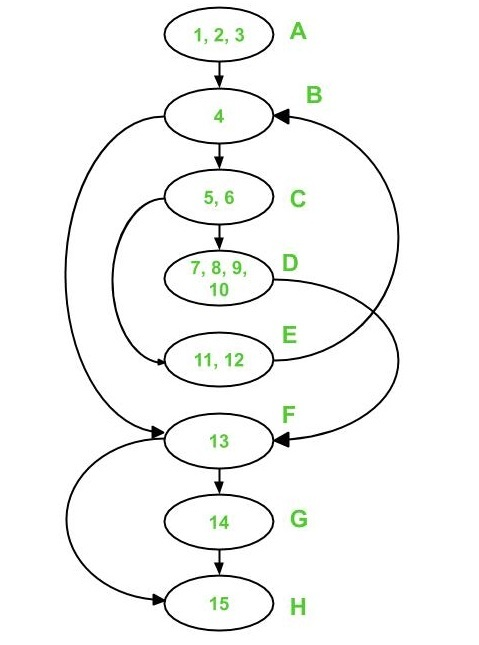2. Calculate the Cyclomatic complexity :

• Method-1:
`V(G) = e - n + 2*p `

In the above control flow graph,

```where, e = 10, n = 8 and p = 1

Therefore,
Cyclomatic Complexity V(G)
= 10 - 8 + 2 * 1
= 4
```
• Method-2:
`V(G) = d + p `

In the above control flow graph,

```where, d = 3 (Node B, C and F) and p = 1

Therefore,
Cyclomatic Complexity V(G)
= 3 + 1
= 4
```
• Method-3:
`V(G) = Number of Regions `

In the above control flow graph, there are 4 regions as shown below :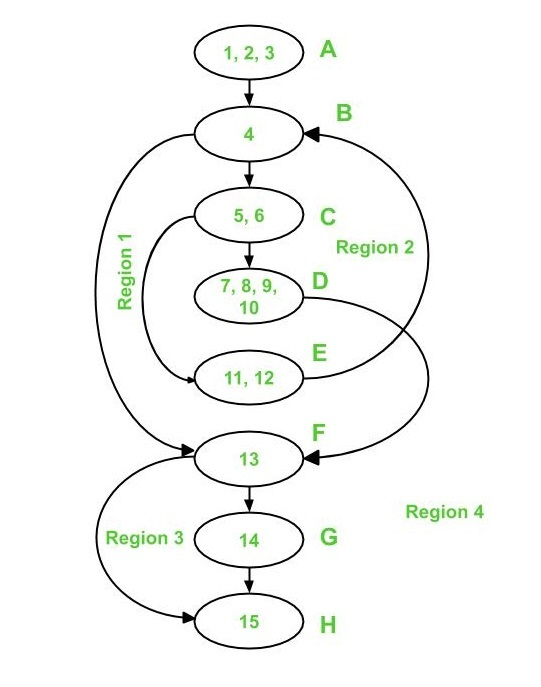```Therefore, there are 4 regions: R1, R2, R3 and R4

Cyclomatic Complexity V(G)
= 1 + 1 + 1 + 1
= 4
```

It is important to note that all three methods give same value for cyclomatic complexity V(G).

3. Independent Paths :
As the cyclomatic complexity V(G) for the graph has come out to be 4, therefore there are 4 independent paths.
Edges covered (marked with red) by Path 1 are:

```Path 1 : A - B - F - G - H
```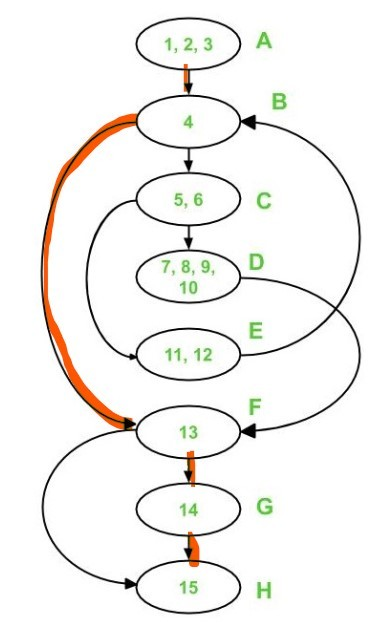Edges covered by Path 1 and Path 2 are shown below :

```Path 2 : A - B - F - H
```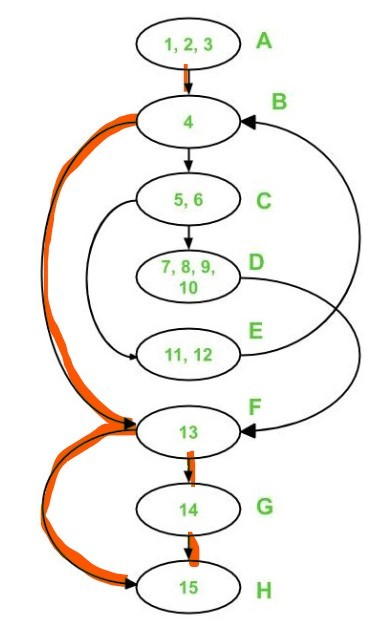Edges covered by Path 1, Path 2 and Path 3 are :

```Path 3 : A - B - C - E - B - F - G - H
```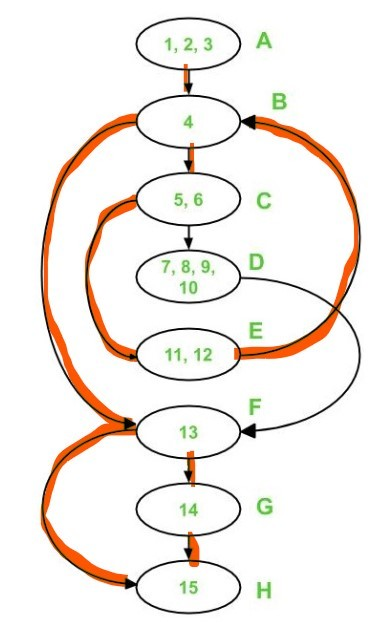Now only 2 edges are left uncovered i.e. edge C-D and edge D-F. Hence, Path 4 must include these two edges.

```Path 4 : A - B - C - D - F - H
```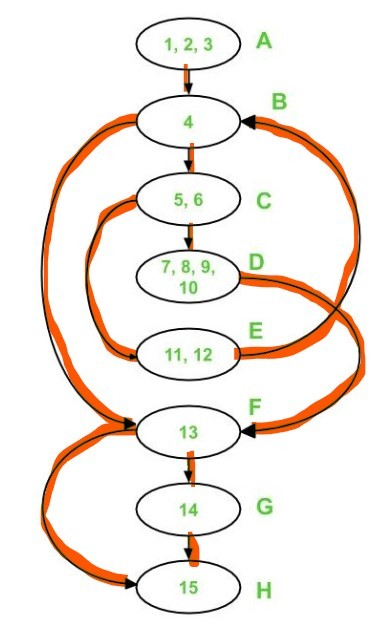Each of these paths have introduced at least one new edge which has not been traversed before.

Note –
Independent paths are not necessarily unique.

4. Test cases :
To derive test cases, we have to use the independent paths obtained previously. To design a test case, provide input to the program such that each independent path is executed.
For the given program, the following test cases will be obtained:

Test case IDInput NumberOutputIndependent Path covered
11No outputA-B-F-H
22It is a prime numberA-B-F-G-H
33It is a prime numberA-B-C-E-B-F-G-H
44It is not a prime numberA-B-C-D-F-H

My Personal Notes arrow_drop_up